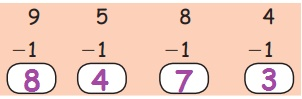Home | | Maths 1st Std | Recall

# Recall

Teacher can ask a few more possible questions following the above example on subtraction concept and elicit the answers from the students.

Unit 2

Numbers

Recall

Observe the picture and answer the following.Total number of fruits in the trees = 9

Total number of birds in the picture = 5

If every bird eats 1 fruit, How many fruits will remain in the trees? 8

Teacher's Note: Teacher can ask a few more possible questions following the above example on subtraction concept and elicit the answers from the students.Subtract

7 −3 = 4

2 −1= 1

5 −4= 1

9 −2= 7

8 −4= 4

3 −1= 2

6 −5= 1

Know more

Subtracting 1 from any number gives its preceding number.9 – 1 = 8

5 – 1 = 4

8 – 1 = 7

4 – 1 = 3

Subtracting the preceding number from any number gives 1.7 – 6 = 1

3 – 2 = 1

6 – 5 = 1

9 – 8 = 1

Tags : Numbers | Term 3 Chapter 2 | 1st Maths , 1st Maths : Term 3 Unit 2 : Numbers
Study Material, Lecturing Notes, Assignment, Reference, Wiki description explanation, brief detail
1st Maths : Term 3 Unit 2 : Numbers : Recall | Numbers | Term 3 Chapter 2 | 1st Maths